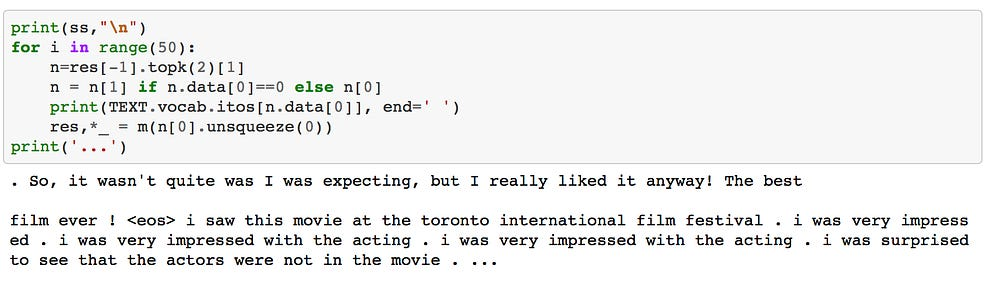Implementing Deep Learning(RNN) in 7 Steps

The Fast.ai community has been known to bring the best cutting-edge technology in the field of Machine Learning/Deep Learning due to its simple implementation which helps to motivate. So, Further, we are going to see how a Language model can be Trained to yield out sentence by its own.

Aim: To Build an automatic Sentence Generator using PyTorch

Step 1:

Data Collection, Cleaning and Data Pre-Processing is the key to achieve state of the art results. It has a huge impact while you train your dataSet.

Step 2:

Split the Data into Two part: a) Train b) Test. Divide them into 80% : 20% ratio percent.
Now import Libraries and Set Path to Dictionary

`from fastai.learner import *`
`import torchtextfrom torchtext import vocab, datafrom torchtext.datasets import language_modeling`
`from fastai.rnn_reg import *from fastai.rnn_train import *from fastai.nlp import *from fastai.lm_rnn import *`
`import dill as pickle`

Now we set Path to the dataset as shown below:

`path = ‘Data/’train_path = ‘Train/’val_path = ‘Test/’`

DataSet has each song in different text Files eg 1.txt, 2.txt and so on.

Note: To check total number of word present in your all Text File, Type unix command:

!find {path}{train_path} -name ‘*.txt’ | xargs cat | wc -w`

Step 3:

To work with Text, first, it’s needed to be converted to a list of words with a total number of word count. That’s called as Tokenization in Natural Language Processing. To do this process, we use spacy_tok() function which is best for tokenization of keywords. Secondly, PyTorch Library has a function called Field() to work with Text as shown below:

`TEXT = data.Field(lower=True, tokenize=spacy_tok)`

Here, lower = True define ensure of the word is in lower case. This is part of TorchText.

Step 4:

Now we will try to create a FastAI Data-Model object. let’s break it down.

`bs = 64 bptt = 70files = dict(train=train_path, validation=val_path, test=val_path)md = LanguageModelData.from_text_files(path,TEXT,train=train_path, validation=val_path,test=val_path,bs=bs,bptt=bptt,min_freq=5)`

Decoding parameter in the above code:

`bs` is known as BatchSize. It’s used to define an array of 64 words which help to input in GPU. If the batch size is more than GPU memory, it would throw an error.

`bptt` Back Propagation through Time defines, how long a sentence will be sticking on GPU at once.

`Dict()` will help to convert it into a dictionary where we pass arguments like training path, validation path & test path. Note we don’t have validation path for this example. So we try to have had the same value for test and validation path.

LanguageModelData.from_text_files() creates language Data model object. here `from_text_file` is basic function used to work with .txt extension for given path. Follows some arguments such as

`Path` = Defined location of Dataset.

`Text` = TorchText preprocessing.

`min_freq` = Defines minimum word count of a single word that should be more than that of defined value. It helps to remove the unwanted word from our dictionary.

Step 5:

Now we would create an embedding Matrix. which is to create all categorical variable which would be defined in a row. and then define layer and hidden activation function in each layer. Then we set Optimizer which is used to help drive to get the best gradient descent. For RNN we generally use ADAM optimizer. More about Recurrent Neural Net Click [here].

`em_sz = 60 # size of each embedding vectornh = 300 # number of hidden activation layernl = 3 # Number of layers`
`# Adam’s Optimizer`
`opt_fn = partial(optim.Adam, betas=(0.7, 0.99))`

Step 6:

Training the data to create an object model which uses AWD LSTM Language model developed recently by Stephen Merity. A key feature is to provide excellent regularization through various dropouts as shown below. Also, we would add regfn function to avoid overfitting plus clip function which would help to reduce the bouncing of gradient descent and find the best value.

`learner = md.get_model(opt_fn, em_sz, nh, nl, dropouti=0.10, dropout=0.10, wdrop=0.2, dropoute=0.03, dropouth=0.10)learner.reg_fn = partial(seq2seq_reg, alpha=2, beta=1)learner.clip=0.3learner.fit(3e-3, 4, wds=1e-6, cycle_len=1, cycle_mult=2)`

Step 7:

Finally, we would arrive at generating words through our trained model. This can be done by as follows:

`print(ss,"\n")for i in range(50):    n=res[-1].topk(2)    n = n if n.data==0 else n    print(TEXT.vocab.itos[n.data], end=' ')    res,*_ = m(n.unsqueeze(0))print('...')`

Result:

This was the easiest way to implement 3 layer Neural Net model using FastAI and PyTorch.

That’s all Folks.

Source: Deep Learning on Medium